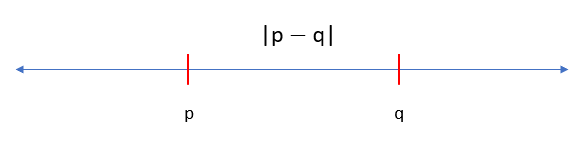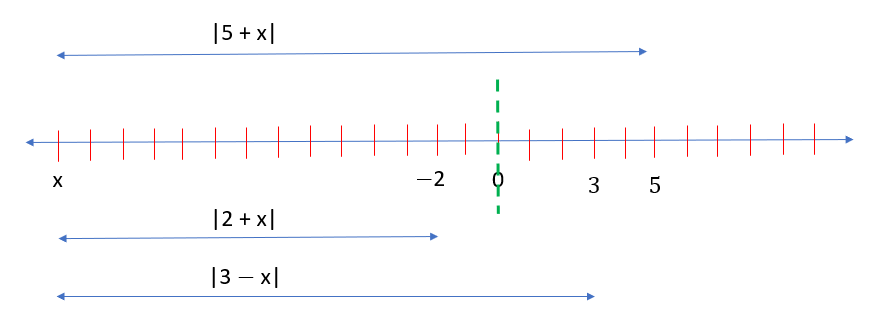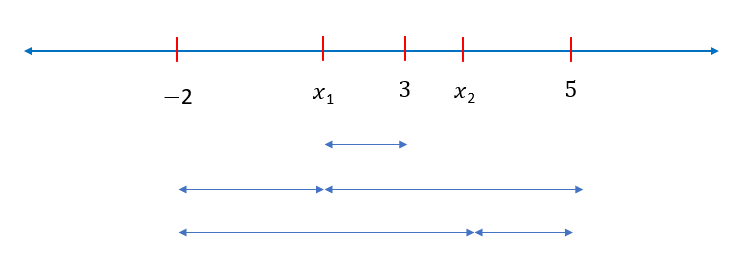# IPMAT Question Paper 2020 | IPM Indore Quants

###### IPMAT Sample Paper | IPMAT Question Paper | Question 5

IPMAT 2020 Question Paper IPM Indore Quantitative Ability. Solve questions from IPMAT 2020 Question Paper from IPM Indore and check the solutions to get adequate practice. The best way to ace IPMAT is by solving IPMAT Question Paper. To solve other IPMAT Sample papers, go here: IPM Sample Paper

Question 5 : The minimum value of f(x)=|3-x|+|2+x|+|5-x| is equal to __________.

## Best CAT Coaching in Chennai

#### CAT Coaching in Chennai - CAT 2022Limited Seats Available - Register Now!The distance between two points of a number line is the difference between the larger point and the smaller point. If the value of the two points p and q are unknown, then the distance is modulus of their difference.

|p - q| = |p - q|

We can re name the points as |x - 3|, |x -(- 2)|, |x - 5|

The points -2 , 3 , 5 need to be marked on the number line.Any point we place on the number line, we calculate the distance from that point to the other points -2,3,5. The minimum value will lie between -2 and 5,because if that point lies outside this range then certain parts between -2 and 5 overlap while calculating the total distance. Hence to be of minimum distance the point has to lie between -2 and 5

Any point between -2 and 5 their sum of distances will add up to 7 .The extra distance will be between the point and 3 which will be minimum when x = 3, because |3 - x| will go to zero

Hence |x - 3| + |x + 2|+ |5 - x|

The minimum distance 0 + 2 + 5 = 7

The question is " The minimum value of f(x)=|3-x|+|2+x|+|5-x| is equal to __________. "

##### Where is 2IIM located?

2IIM Online CAT Coaching
A Fermat Education Initiative,
58/16, Indira Gandhi Street,
Kaveri Rangan Nagar, Saligramam, Chennai 600 093

##### How to reach 2IIM?

Mobile: (91) 99626 48484 / 94459 38484
WhatsApp: WhatsApp Now
Email: info@2iim.com